Скачать презентацию Interaction Control Manipulation requires interaction object

e1593d35ab1ecb698d8331949e1197f9.ppt

• Количество слайдов: 35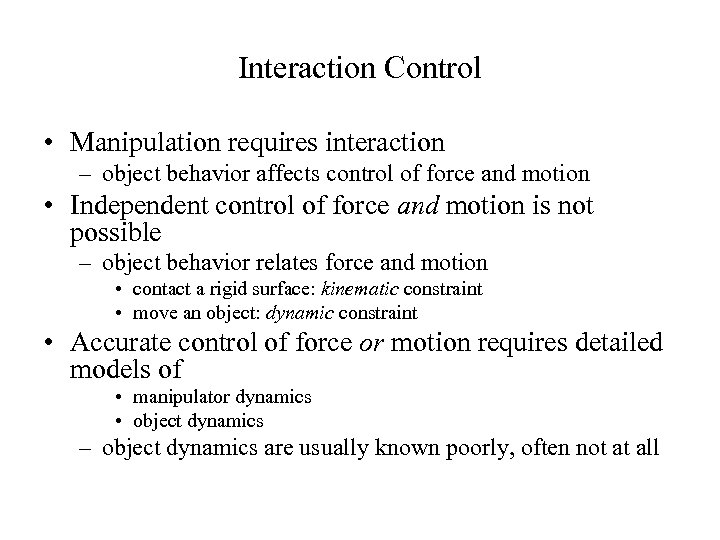Interaction Control • Manipulation requires interaction – object behavior affects control of force and motion • Independent control of force and motion is not possible – object behavior relates force and motion • contact a rigid surface: kinematic constraint • move an object: dynamic constraint • Accurate control of force or motion requires detailed models of • manipulator dynamics • object dynamics – object dynamics are usually known poorly, often not at all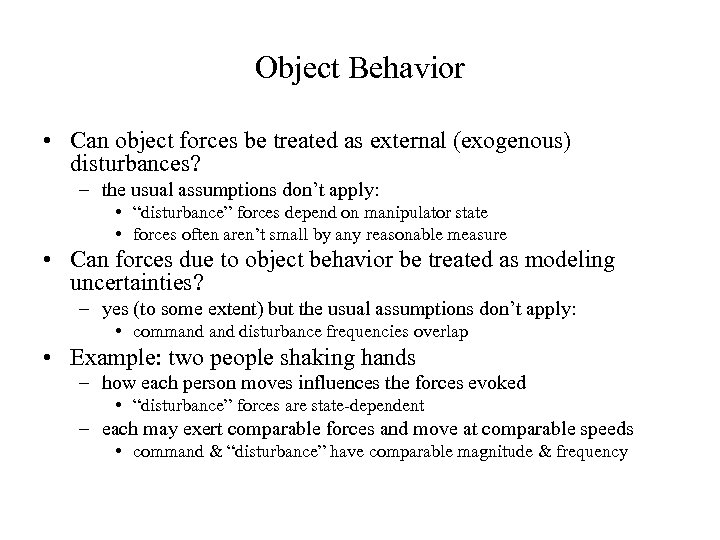Object Behavior • Can object forces be treated as external (exogenous) disturbances? – the usual assumptions don’t apply: • “disturbance” forces depend on manipulator state • forces often aren’t small by any reasonable measure • Can forces due to object behavior be treated as modeling uncertainties? – yes (to some extent) but the usual assumptions don’t apply: • command disturbance frequencies overlap • Example: two people shaking hands – how each person moves influences the forces evoked • “disturbance” forces are state-dependent – each may exert comparable forces and move at comparable speeds • command & “disturbance” have comparable magnitude & frequency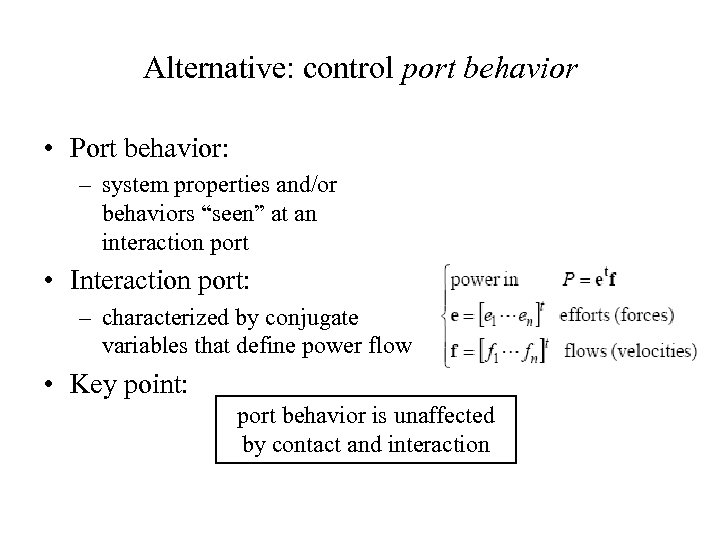Alternative: control port behavior • Port behavior: – system properties and/or behaviors “seen” at an interaction port • Interaction port: – characterized by conjugate variables that define power flow • Key point: port behavior is unaffected by contact and interaction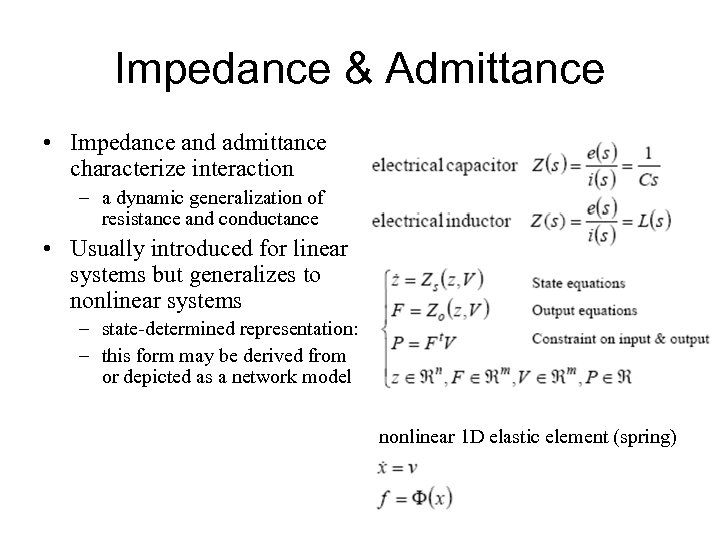Impedance & Admittance • Impedance and admittance characterize interaction – a dynamic generalization of resistance and conductance • Usually introduced for linear systems but generalizes to nonlinear systems – state-determined representation: – this form may be derived from or depicted as a network model nonlinear 1 D elastic element (spring)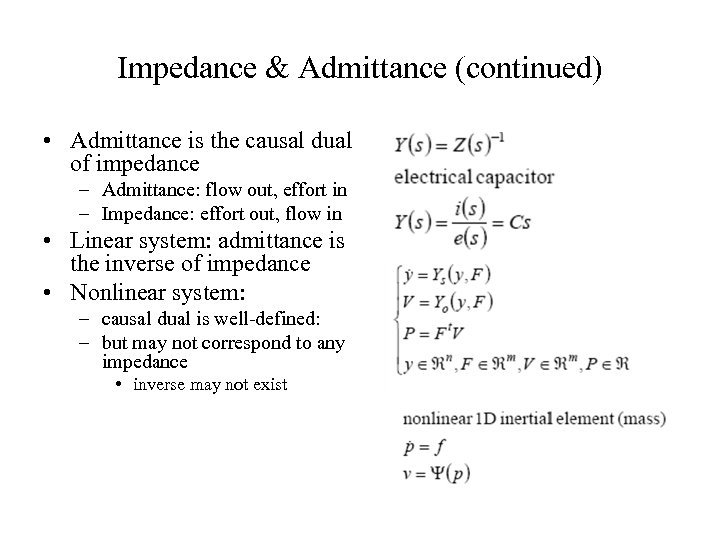Impedance & Admittance (continued) • Admittance is the causal dual of impedance – Admittance: flow out, effort in – Impedance: effort out, flow in • Linear system: admittance is the inverse of impedance • Nonlinear system: – causal dual is well-defined: – but may not correspond to any impedance • inverse may not exist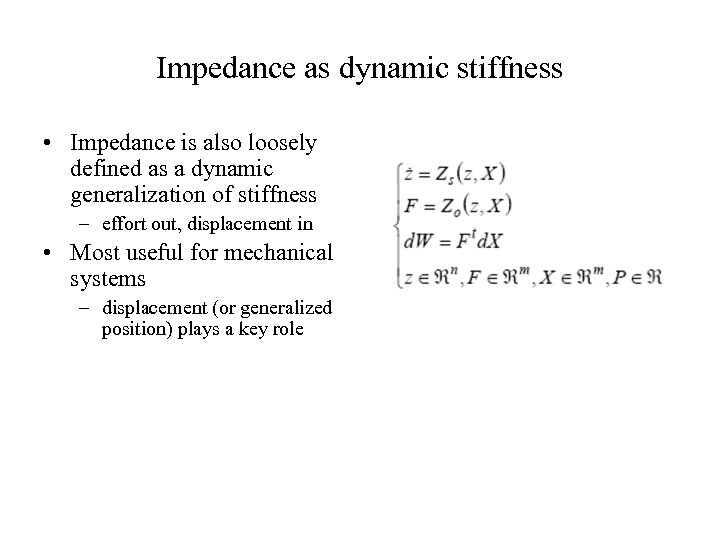Impedance as dynamic stiffness • Impedance is also loosely defined as a dynamic generalization of stiffness – effort out, displacement in • Most useful for mechanical systems – displacement (or generalized position) plays a key role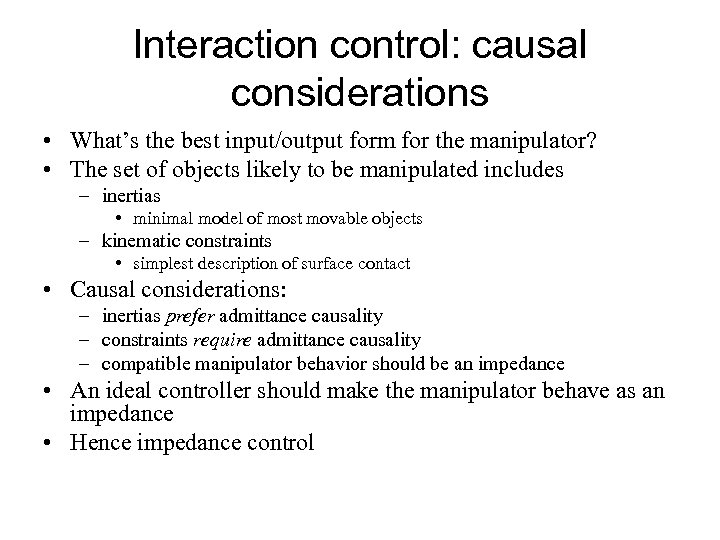Interaction control: causal considerations • What’s the best input/output form for the manipulator? • The set of objects likely to be manipulated includes – inertias • minimal model of most movable objects – kinematic constraints • simplest description of surface contact • Causal considerations: – inertias prefer admittance causality – constraints require admittance causality – compatible manipulator behavior should be an impedance • An ideal controller should make the manipulator behave as an impedance • Hence impedance control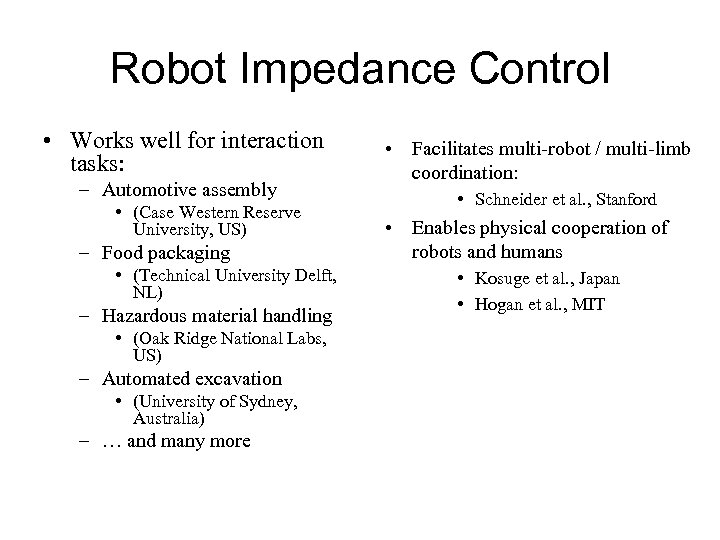Robot Impedance Control • Works well for interaction tasks: – Automotive assembly • (Case Western Reserve University, US) – Food packaging • (Technical University Delft, NL) – Hazardous material handling • (Oak Ridge National Labs, US) – Automated excavation • (University of Sydney, Australia) – … and many more • Facilitates multi-robot / multi-limb coordination: • Schneider et al. , Stanford • Enables physical cooperation of robots and humans • Kosuge et al. , Japan • Hogan et al. , MIT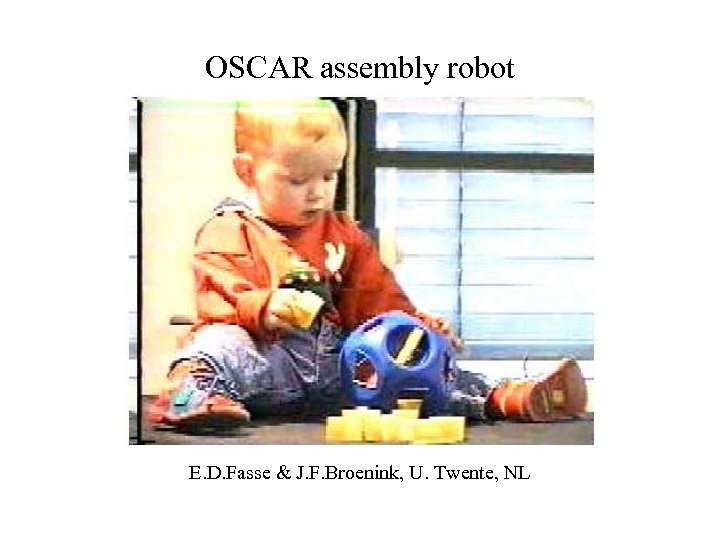OSCAR assembly robot E. D. Fasse & J. F. Broenink, U. Twente, NL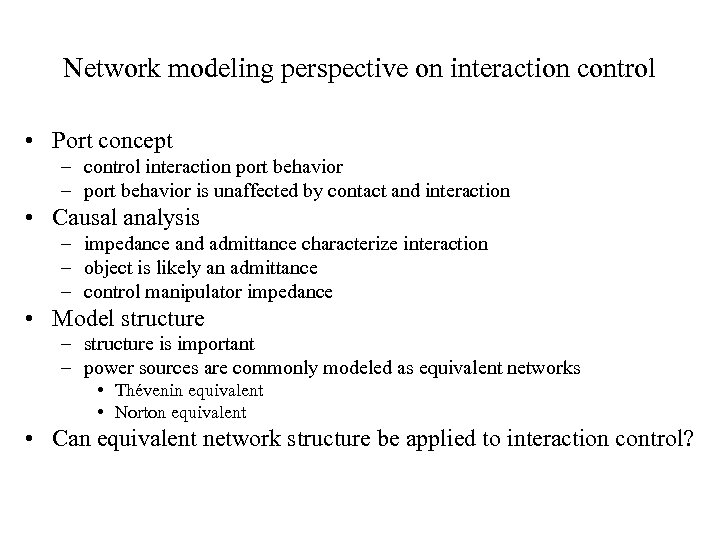Network modeling perspective on interaction control • Port concept – control interaction port behavior – port behavior is unaffected by contact and interaction • Causal analysis – impedance and admittance characterize interaction – object is likely an admittance – control manipulator impedance • Model structure – structure is important – power sources are commonly modeled as equivalent networks • Thévenin equivalent • Norton equivalent • Can equivalent network structure be applied to interaction control?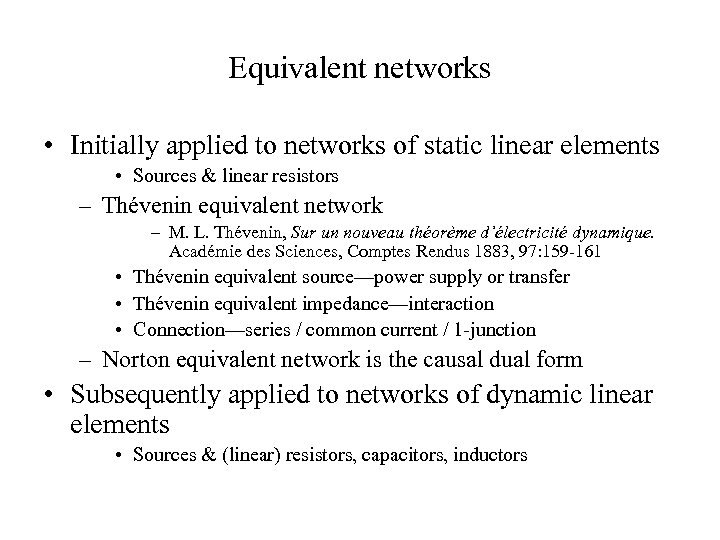Equivalent networks • Initially applied to networks of static linear elements • Sources & linear resistors – Thévenin equivalent network – M. L. Thévenin, Sur un nouveau théorème d’électricité dynamique. Académie des Sciences, Comptes Rendus 1883, 97: 159 -161 • Thévenin equivalent source—power supply or transfer • Thévenin equivalent impedance—interaction • Connection—series / common current / 1 -junction – Norton equivalent network is the causal dual form • Subsequently applied to networks of dynamic linear elements • Sources & (linear) resistors, capacitors, inductors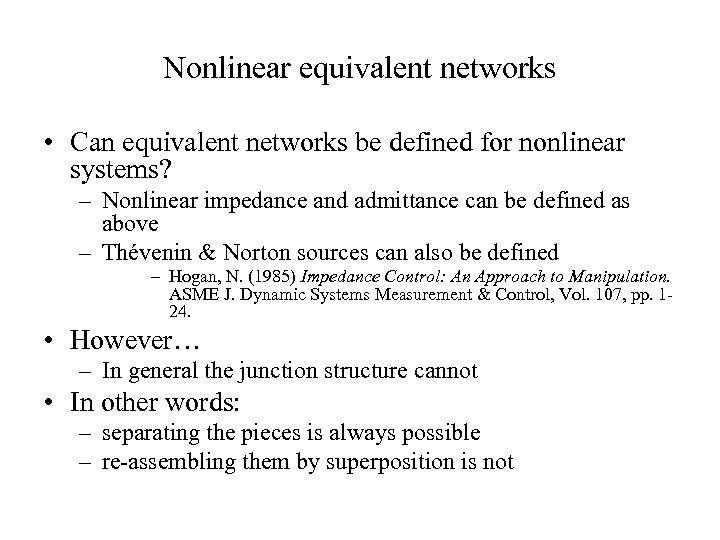Nonlinear equivalent networks • Can equivalent networks be defined for nonlinear systems? – Nonlinear impedance and admittance can be defined as above – Thévenin & Norton sources can also be defined – Hogan, N. (1985) Impedance Control: An Approach to Manipulation. ASME J. Dynamic Systems Measurement & Control, Vol. 107, pp. 124. • However… – In general the junction structure cannot • In other words: – separating the pieces is always possible – re-assembling them by superposition is not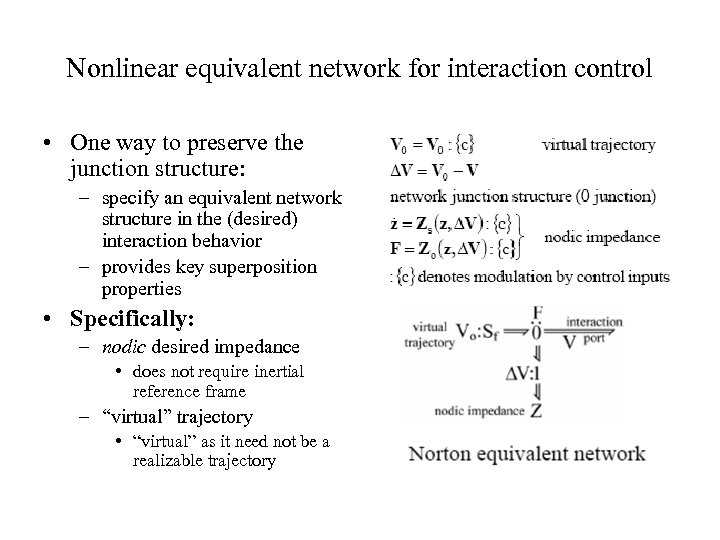Nonlinear equivalent network for interaction control • One way to preserve the junction structure: – specify an equivalent network structure in the (desired) interaction behavior – provides key superposition properties • Specifically: – nodic desired impedance • does not require inertial reference frame – “virtual” trajectory • “virtual” as it need not be a realizable trajectory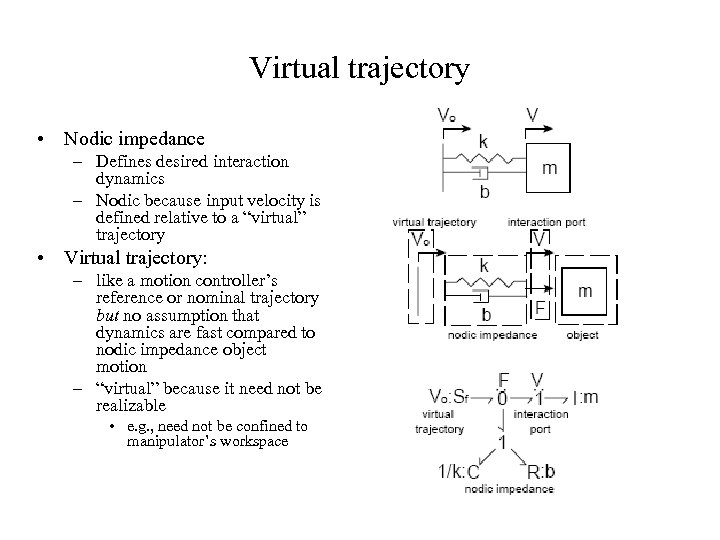Virtual trajectory • Nodic impedance – Defines desired interaction dynamics – Nodic because input velocity is defined relative to a “virtual” trajectory • Virtual trajectory: – like a motion controller’s reference or nominal trajectory but no assumption that dynamics are fast compared to nodic impedance object motion – “virtual” because it need not be realizable • e. g. , need not be confined to manipulator’s workspace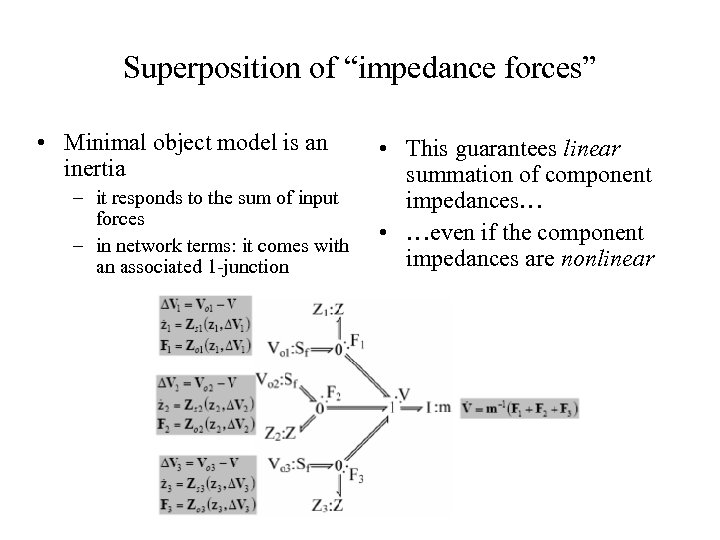Superposition of “impedance forces” • Minimal object model is an inertia – it responds to the sum of input forces – in network terms: it comes with an associated 1 -junction • This guarantees linear summation of component impedances… • …even if the component impedances are nonlinear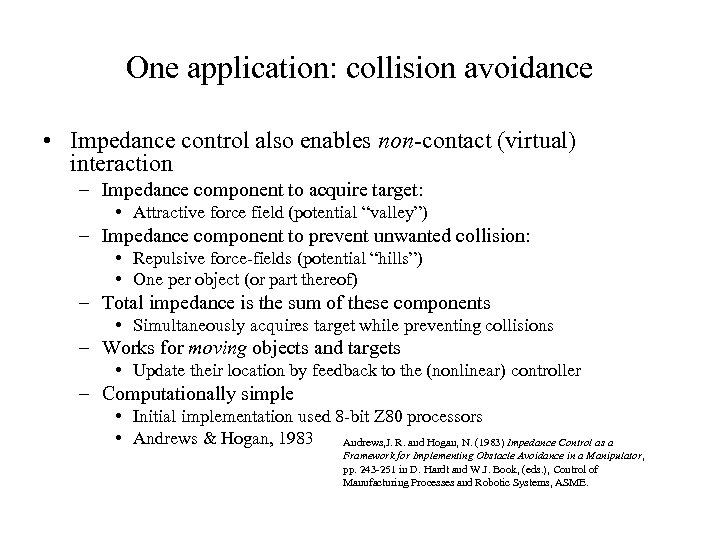One application: collision avoidance • Impedance control also enables non-contact (virtual) interaction – Impedance component to acquire target: • Attractive force field (potential “valley”) – Impedance component to prevent unwanted collision: • Repulsive force-fields (potential “hills”) • One per object (or part thereof) – Total impedance is the sum of these components • Simultaneously acquires target while preventing collisions – Works for moving objects and targets • Update their location by feedback to the (nonlinear) controller – Computationally simple • Initial implementation used 8 -bit Z 80 processors • Andrews & Hogan, 1983 Andrews, J. R. and Hogan, N. (1983) Impedance Control as a Framework for Implementing Obstacle Avoidance in a Manipulator, pp. 243 -251 in D. Hardt and W. J. Book, (eds. ), Control of Manufacturing Processes and Robotic Systems, ASME.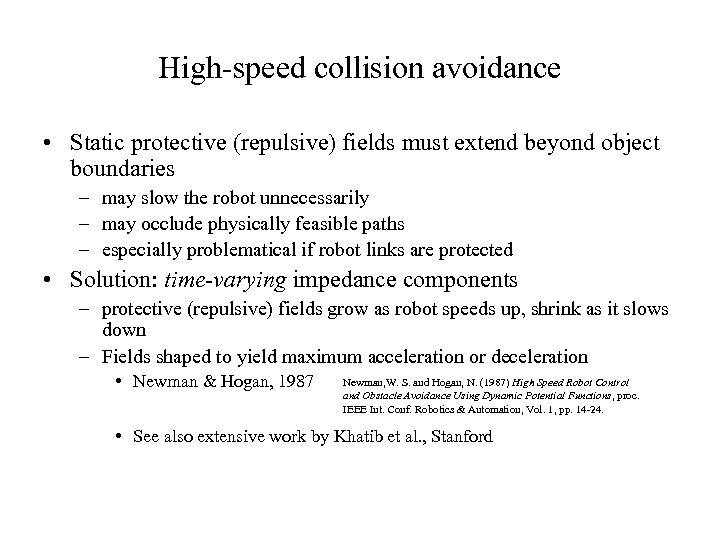High-speed collision avoidance • Static protective (repulsive) fields must extend beyond object boundaries – may slow the robot unnecessarily – may occlude physically feasible paths – especially problematical if robot links are protected • Solution: time-varying impedance components – protective (repulsive) fields grow as robot speeds up, shrink as it slows down – Fields shaped to yield maximum acceleration or deceleration • Newman & Hogan, 1987 Newman, W. S. and Hogan, N. (1987) High Speed Robot Control and Obstacle Avoidance Using Dynamic Potential Functions, proc. IEEE Int. Conf. Robotics & Automation, Vol. 1, pp. 14 -24. • See also extensive work by Khatib et al. , Stanford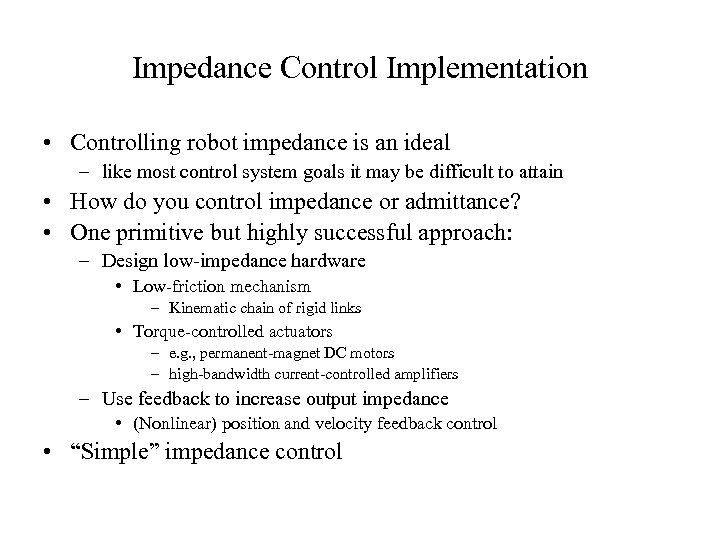Impedance Control Implementation • Controlling robot impedance is an ideal – like most control system goals it may be difficult to attain • How do you control impedance or admittance? • One primitive but highly successful approach: – Design low-impedance hardware • Low-friction mechanism – Kinematic chain of rigid links • Torque-controlled actuators – e. g. , permanent-magnet DC motors – high-bandwidth current-controlled amplifiers – Use feedback to increase output impedance • (Nonlinear) position and velocity feedback control • “Simple” impedance control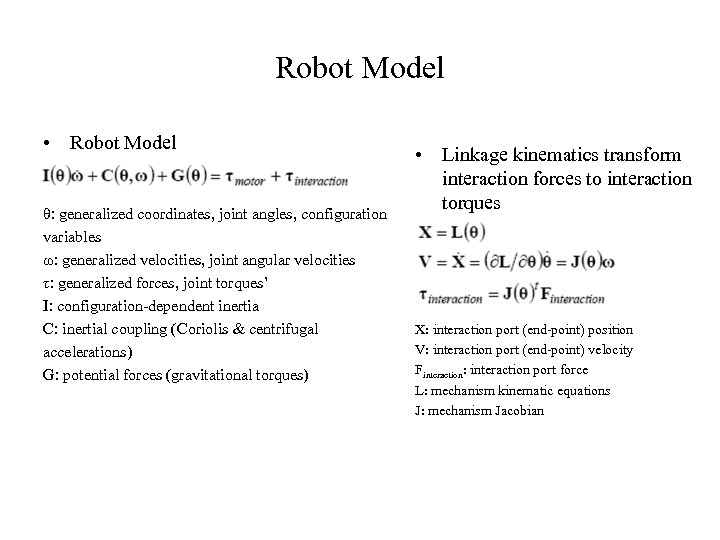Robot Model • Robot Model θ: generalized coordinates, joint angles, configuration variables ω: generalized velocities, joint angular velocities τ: generalized forces, joint torques’ I: configuration-dependent inertia C: inertial coupling (Coriolis & centrifugal accelerations) G: potential forces (gravitational torques) • Linkage kinematics transform interaction forces to interaction torques X: interaction port (end-point) position V: interaction port (end-point) velocity Finteraction : interaction port force L: mechanism kinematic equations J: mechanism Jacobian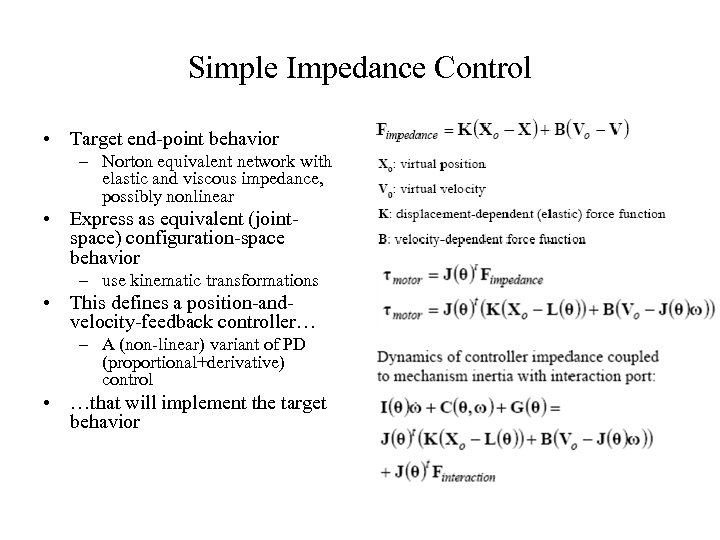Simple Impedance Control • Target end-point behavior – Norton equivalent network with elastic and viscous impedance, possibly nonlinear • Express as equivalent (jointspace) configuration-space behavior – use kinematic transformations • This defines a position-andvelocity-feedback controller… – A (non-linear) variant of PD (proportional+derivative) control • …that will implement the target behavior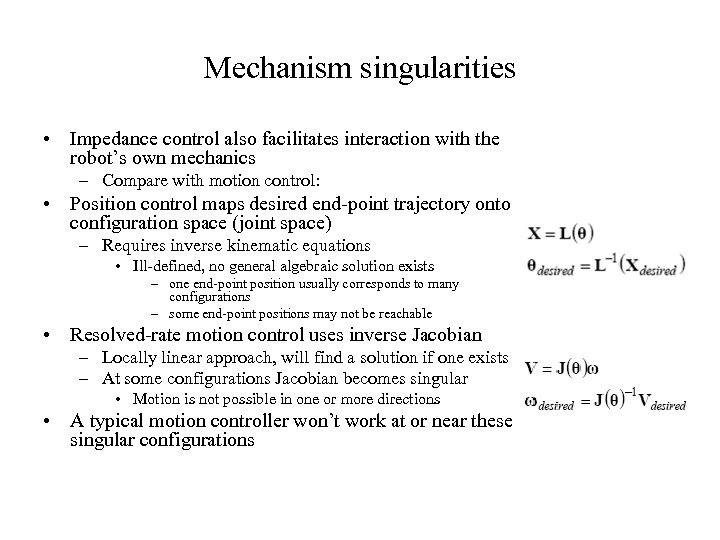Mechanism singularities • Impedance control also facilitates interaction with the robot’s own mechanics – Compare with motion control: • Position control maps desired end-point trajectory onto configuration space (joint space) – Requires inverse kinematic equations • Ill-defined, no general algebraic solution exists – one end-point position usually corresponds to many configurations – some end-point positions may not be reachable • Resolved-rate motion control uses inverse Jacobian – Locally linear approach, will find a solution if one exists – At some configurations Jacobian becomes singular • Motion is not possible in one or more directions • A typical motion controller won’t work at or near these singular configurations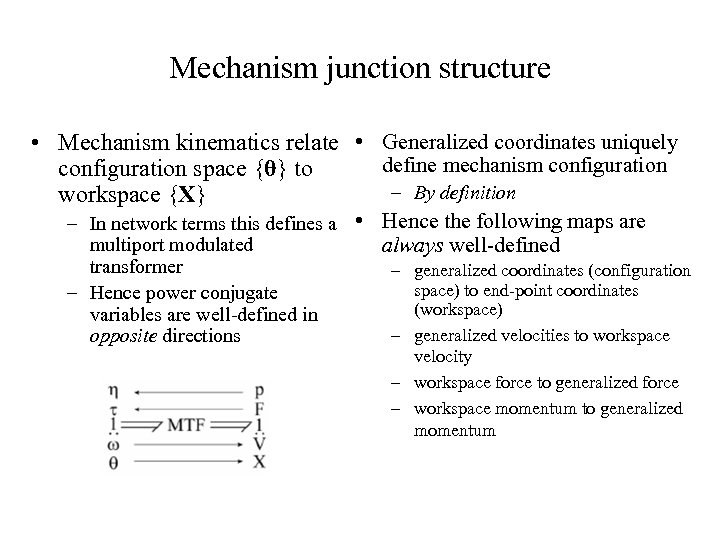Mechanism junction structure • Mechanism kinematics relate • Generalized coordinates uniquely define mechanism configuration space {θ} to – By definition workspace {X} – In network terms this defines a • Hence the following maps are multiport modulated always well-defined transformer – generalized coordinates (configuration space) to end-point coordinates – Hence power conjugate (workspace) variables are well-defined in – generalized velocities to workspace opposite directions velocity – workspace force to generalized force – workspace momentum to generalized momentum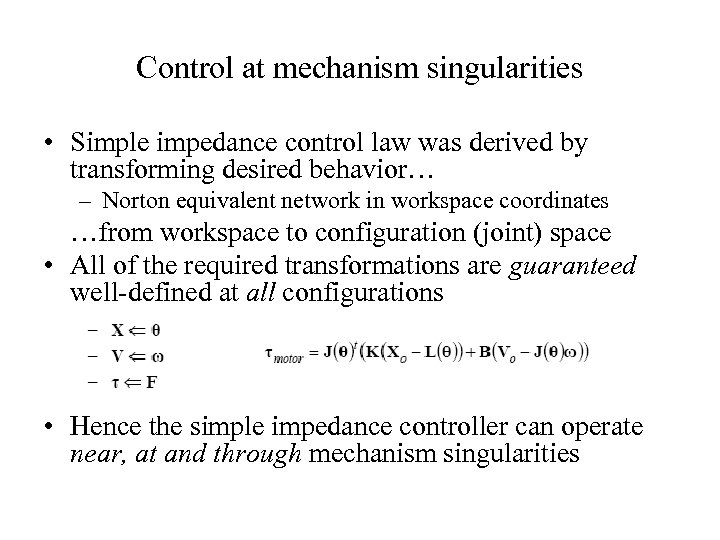Control at mechanism singularities • Simple impedance control law was derived by transforming desired behavior… – Norton equivalent network in workspace coordinates …from workspace to configuration (joint) space • All of the required transformations are guaranteed well-defined at all configurations • Hence the simple impedance controller can operate near, at and through mechanism singularities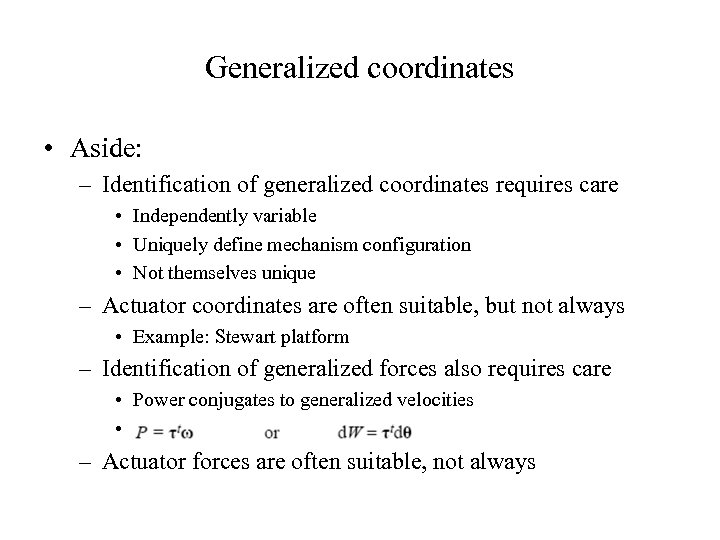Generalized coordinates • Aside: – Identification of generalized coordinates requires care • Independently variable • Uniquely define mechanism configuration • Not themselves unique – Actuator coordinates are often suitable, but not always • Example: Stewart platform – Identification of generalized forces also requires care • Power conjugates to generalized velocities • – Actuator forces are often suitable, not always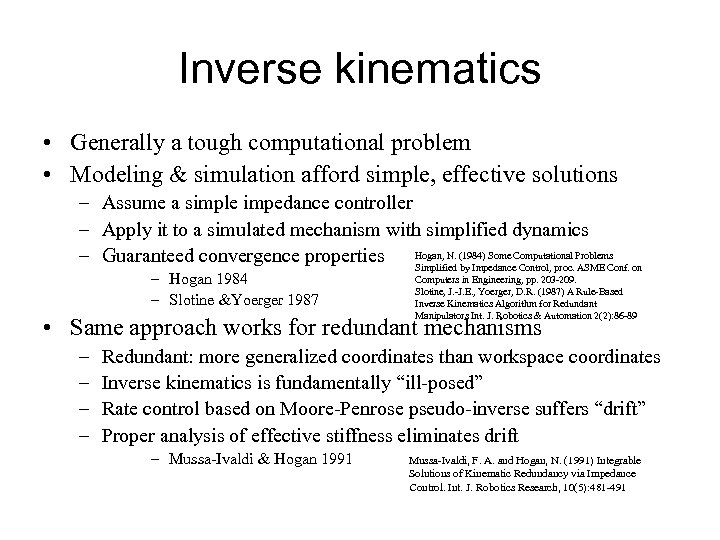Inverse kinematics • Generally a tough computational problem • Modeling & simulation afford simple, effective solutions – Assume a simple impedance controller – Apply it to a simulated mechanism with simplified dynamics – Guaranteed convergence properties Hogan, N. (1984) Some Computational Problems on Simplified by Impedance Control, proc. ASME Conf. – Hogan 1984 – Slotine &Yoerger 1987 Computers in Engineering, pp. 203 -209. Slotine, J. -J. E. , Yoerger, D. R. (1987) A Rule-Based Inverse Kinematics Algorithm for Redundant Manipulators Int. J. Robotics & Automation 2(2): 86 -89 • Same approach works for redundant mechanisms – – Redundant: more generalized coordinates than workspace coordinates Inverse kinematics is fundamentally “ill-posed” Rate control based on Moore-Penrose pseudo-inverse suffers “drift” Proper analysis of effective stiffness eliminates drift – Mussa-Ivaldi & Hogan 1991 Mussa-Ivaldi, F. A. and Hogan, N. (1991) Integrable Solutions of Kinematic Redundancy via Impedance Control. Int. J. Robotics Research, 10(5): 481 -491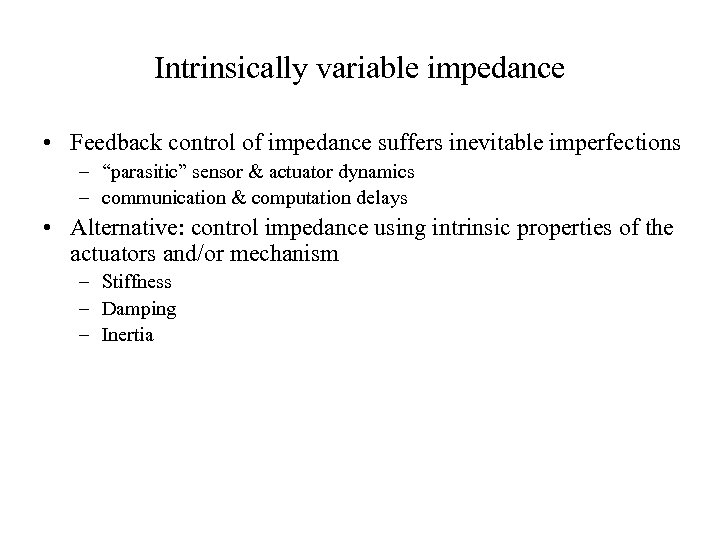Intrinsically variable impedance • Feedback control of impedance suffers inevitable imperfections – “parasitic” sensor & actuator dynamics – communication & computation delays • Alternative: control impedance using intrinsic properties of the actuators and/or mechanism – Stiffness – Damping – Inertia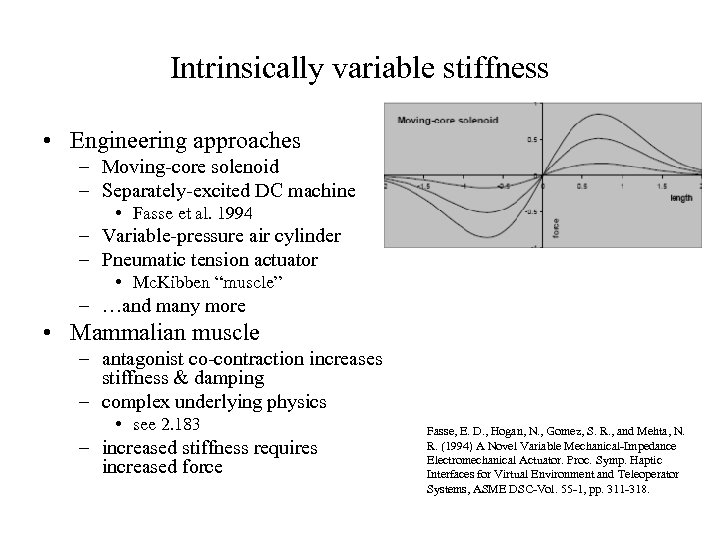Intrinsically variable stiffness • Engineering approaches – Moving-core solenoid – Separately-excited DC machine • Fasse et al. 1994 – Variable-pressure air cylinder – Pneumatic tension actuator • Mc. Kibben “muscle” – …and many more • Mammalian muscle – antagonist co-contraction increases stiffness & damping – complex underlying physics • see 2. 183 – increased stiffness requires increased force Fasse, E. D. , Hogan, N. , Gomez, S. R. , and Mehta, N. R. (1994) A Novel Variable Mechanical-Impedance Electromechanical Actuator. Proc. Symp. Haptic Interfaces for Virtual Environment and Teleoperator Systems, ASME DSC-Vol. 55 -1, pp. 311 -318.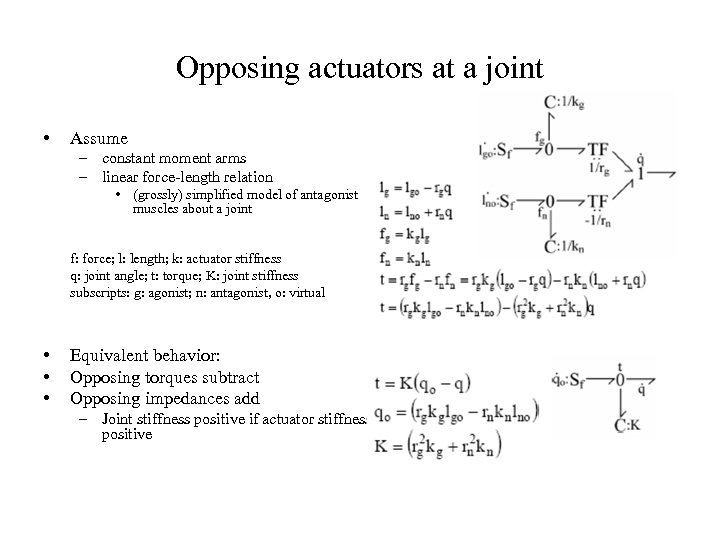Opposing actuators at a joint • Assume – constant moment arms – linear force-length relation • (grossly) simplified model of antagonist muscles about a joint f: force; l: length; k: actuator stiffness q: joint angle; t: torque; K: joint stiffness subscripts: g: agonist; n: antagonist, o: virtual • • • Equivalent behavior: Opposing torques subtract Opposing impedances add – Joint stiffness positive if actuator stiffness positive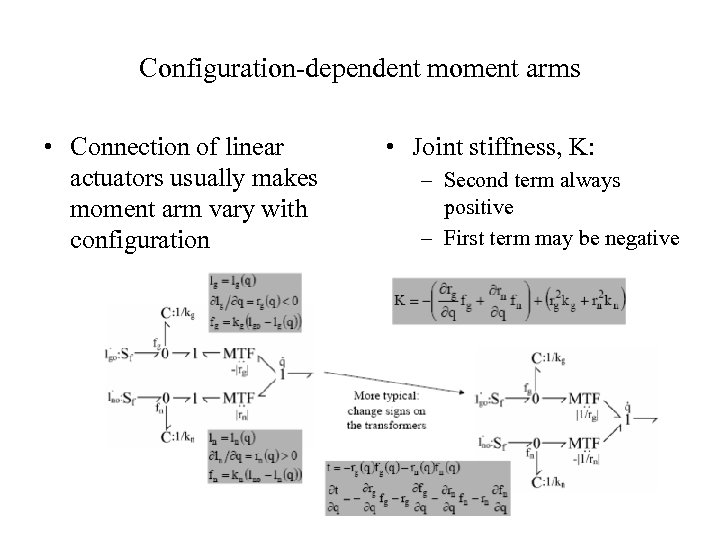Configuration-dependent moment arms • Connection of linear actuators usually makes moment arm vary with configuration • Joint stiffness, K: – Second term always positive – First term may be negative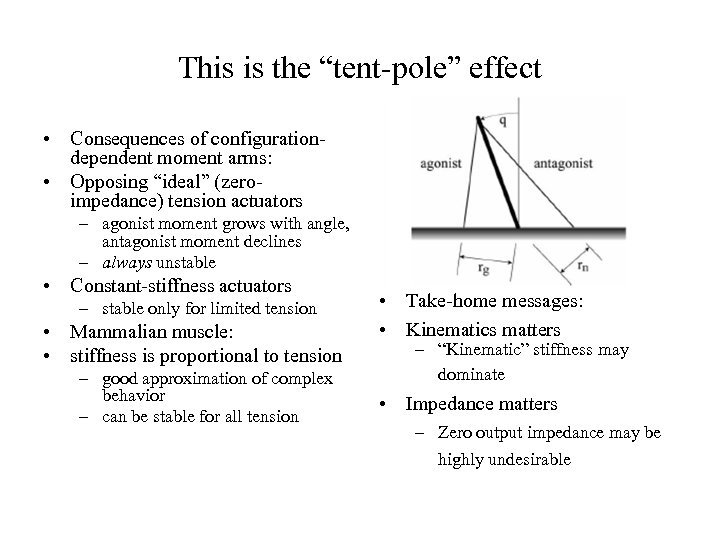This is the “tent-pole” effect • Consequences of configurationdependent moment arms: • Opposing “ideal” (zeroimpedance) tension actuators – agonist moment grows with angle, antagonist moment declines – always unstable • Constant-stiffness actuators – stable only for limited tension • Mammalian muscle: • stiffness is proportional to tension – good approximation of complex behavior – can be stable for all tension • Take-home messages: • Kinematics matters – “Kinematic” stiffness may dominate • Impedance matters – Zero output impedance may be highly undesirable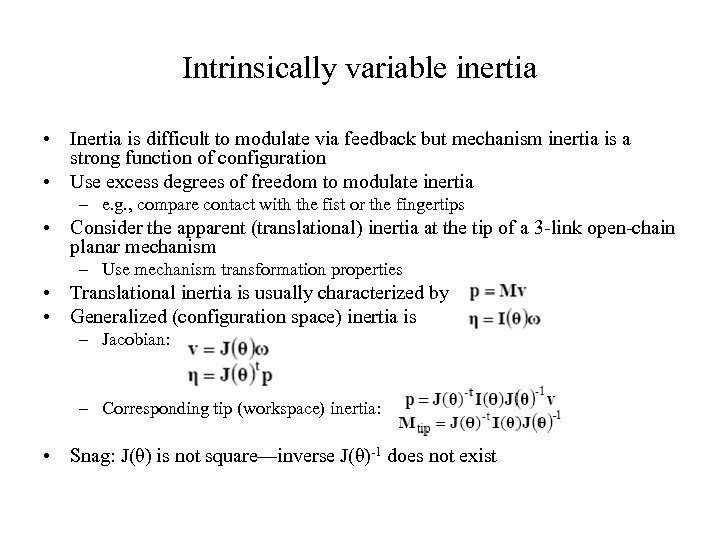Intrinsically variable inertia • Inertia is difficult to modulate via feedback but mechanism inertia is a strong function of configuration • Use excess degrees of freedom to modulate inertia – e. g. , compare contact with the fist or the fingertips • Consider the apparent (translational) inertia at the tip of a 3 -link open-chain planar mechanism – Use mechanism transformation properties • Translational inertia is usually characterized by • Generalized (configuration space) inertia is – Jacobian: – Corresponding tip (workspace) inertia: • Snag: J(θ) is not square—inverse J(θ)-1 does not exist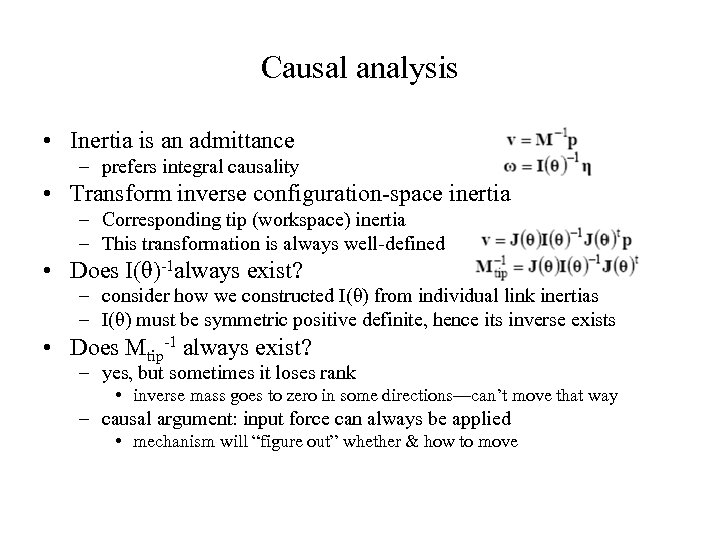Causal analysis • Inertia is an admittance – prefers integral causality • Transform inverse configuration-space inertia – Corresponding tip (workspace) inertia – This transformation is always well-defined • Does I(θ)-1 always exist? – consider how we constructed I(θ) from individual link inertias – I(θ) must be symmetric positive definite, hence its inverse exists • Does Mtip-1 always exist? – yes, but sometimes it loses rank • inverse mass goes to zero in some directions—can’t move that way – causal argument: input force can always be applied • mechanism will “figure out” whether & how to moveAppendix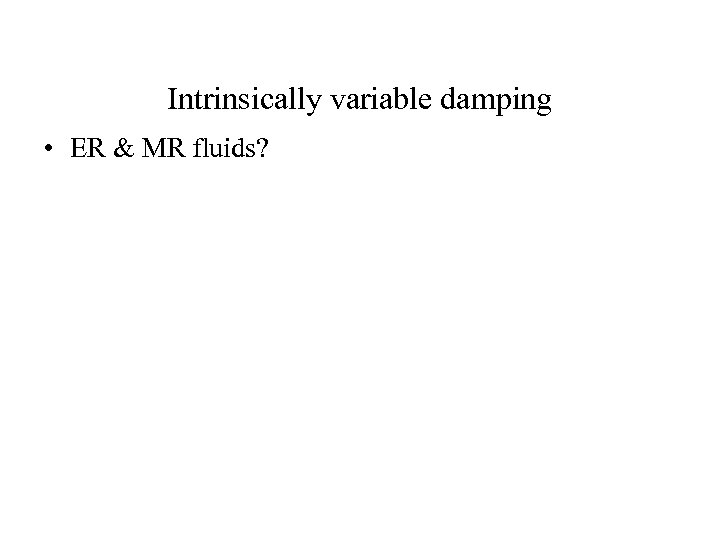Intrinsically variable damping • ER & MR fluids?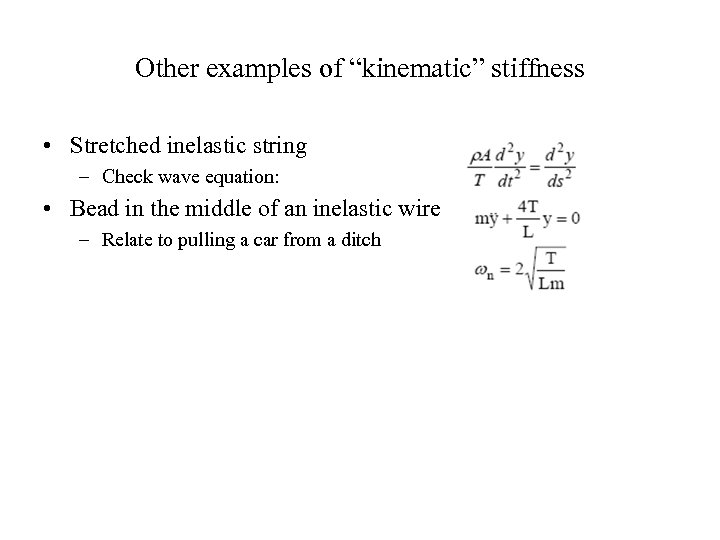Other examples of “kinematic” stiffness • Stretched inelastic string – Check wave equation: • Bead in the middle of an inelastic wire – Relate to pulling a car from a ditch